## Example Questions

### Example Question #1 : Consecutive Integers

What is the sum of all of the four-digit integers that can be created with the digits 1, 2, 3, and 4?

482,912

37,891

711,040

5994

48,758

711,040

Explanation:

First we need to find out how many possible numbers there are. The number of possible four-digit numbers with four different digits is simply 4 * 4 * 4 * 4 = 256.

To find the sum, the formula we must remember is sum = average * number of values. The last piece that's missing in this formula is the average. To find this, we can average the first and last number, since the numbers are consecutive. The smallest number that can be created from 1, 2, 3, and 4 is 1111, and the largest number possible is 4444. Then the average is (1111 + 4444)/2.

So sum = 5555/2 * 256 = 711,040.

### Example Question #1 : Consecutive Integers

Quantity A: The sum of all integers from 49 to 98 inclusive.

Quantity B: The sum of all integers from 51 to 99 inclusive.

Quantity A is greater.

Quantity B is greater.

The relationship cannot be determined from the information given.

The two quantities are equal.

The two quantities are equal.

Explanation:

For each quantity, only count the integers that aren't in the other quantity. Both quantities include the numbers 51 to 98, so those numbers won't affect which is greater. Only Quantity A has 49 and 50 (for a total of 99) and only Quantity B has 99. Since the excluded numbers from both quantities equal 99, you can conclude that the 2 quantities are equal.

### Example Question #3 : Consecutive Integers

What is the sum of all the integers between 1 and 69, inclusive?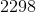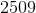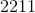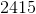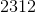Explanation:

The formula here is sum = average value * number of values.  Since this is a consecutive series, the average can be found by averaging only the first and last terms: (1 + 69)/2 = 35.

sum = average * number of values = 35 * 69 = 2415

### Example Question #4 : Consecutive Integers

Quantity A: The sum of all integers from 1 to 30

Quantity B: 465

The relationship cannot be determined from the information given.

Quantity B is greater.

The two quantities are equal.

Quantity A is greater.

The two quantities are equal.

Explanation:

The sum of all integers from 1 to 30 can be found using the formula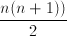, where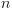is 30. In this case, the sum equals 465.

### Example Question #5 : Consecutive Integers

The sum of a set ofconsecutive odd integers is.  What is the fourth number in this set?Explanation:

We can represent our numbers as: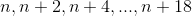will have to be an odd number since the whole sequence is odd.  However, this will work out when we do the math.  Now, we know that all of these added up will be.  We have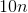and the sum of the set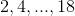, the sum of which is.

Thus, we know: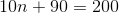Solve for: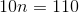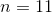Therefore, the fourth element will be: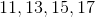### Example Question #6 : Consecutive Integers

The average of five consecutive integers is.  What is the largest of these integers?Explanation:

There are two ways to figure out this list of integers.  On the one hand, you might know that the average of a set of consecutive integers is the "middle value" of that set.  So, if the average isand the size, the list must be: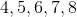Another way to figure this out is to represent your integers as: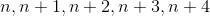The average of these values will be all of these numbers added together and then divided by.  This gives us: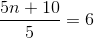Multiply both sides by: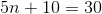Finish solving: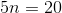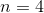This means that the largest value is: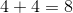### Example Question #7 : Consecutive Integers

If the sum of four consecutive numbers is 38, what is the mean of the largest and the smallest of the four numbers?

8.75

9.5

10.5

11

11.75

9.5

Explanation:

The sum of 4 consecutive numbers being 38 can be written as the following equation.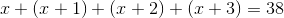We can simplify this to solve for x.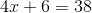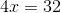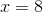This tells us that the smallest number is 8 and the largest is (8 + 3) = 11. From this, we can find their mean.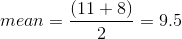Tired of practice problems?

Try live online GRE prep today.